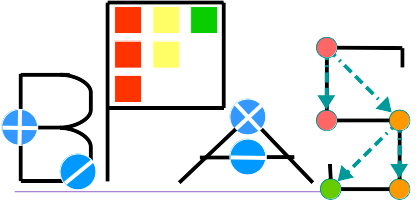## Targeted functionalities

### Level 1: core routines specific to a coefficient ring or a polynomial representation

• Code is highly optimized in terms of work, data locality and parallelism.
• Automatic code generation is used at library installation time.
• Example: one-dimensional FFT

### Level 2: basic arithmetic operations

• Functions provide a variety of algorithmic solutions for a given operation.
• The user can choose between algorithms minimizing work or algorithms maximizing parallelism.
• Example: Schönaghe-Strassen, divide-and-conquer, k-way Toom-Cook and the two-convolution method for integer polynomial multiplication

### Level 3: advanced arithmetic operations

• Functions combine several Level 2 algorithms for achieving a given task.
• This leads to adaptive algorithms that select appropriate Level 2 functions depending on available resources (number of cores, input data size).
• Example: Taylor shift operation

## A snapshot of the BPAS ring classes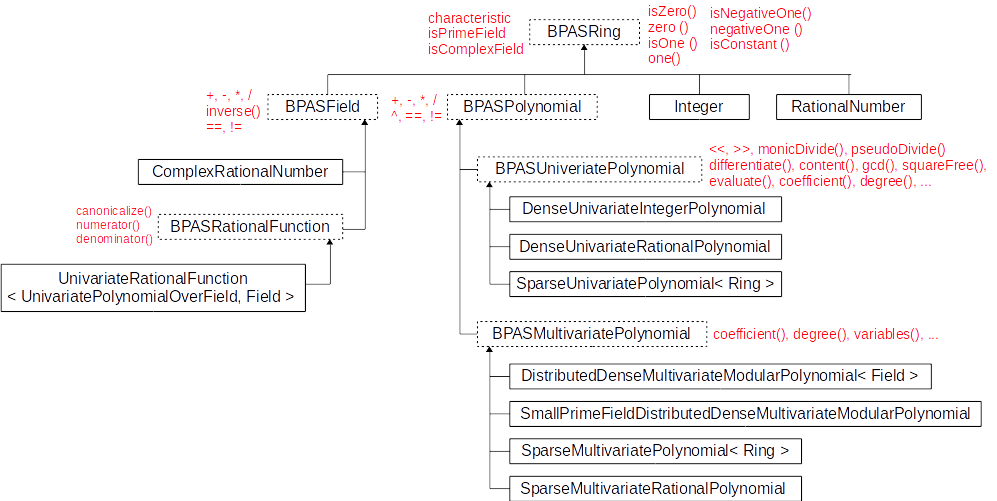• The BPAS classes Integer and RationalNumber are BPAS wrappers for GMP's mpz and mpq classes.
• Many other classes are provided, like Intervals, RationalRegularChain, ...

## One-dimensional modular FFTs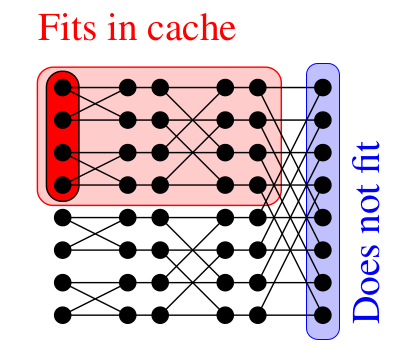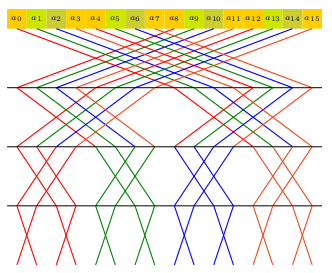• Assuming an ideal cache of Z words with L words per cache line; n be the input vector size
• Traditional 2-way FFT proceeds on the FFT graph row-by-row (left picture above) and a cache complexity in Ω(n / L (log2(n) - log2(Z))), which is not optimal.
• By proceeding in a block-wise manner (picture on the right), cache complexity becomes Ο(n / L (log2(n) / log2(Z))), which is optimal.
• BPAS 1-D FFT code is automatically generated by a configurable Python script implementing cache-optimal FFTs.
• In addition to the optimal blocking strategy, instruction level parallelism (ILP) is carefully considered: vectorized instructions are explicitly used (SSE2, SSE4) and instruction pipeline usage is highly optimized.
• Experimental results: Modpn (serial) vs BPAS (serial)## Parallel multiplication of dense integer polynomials

• An adaptive algorithm based on the input size and available cores
• Very small: Plain multiplication
• Small or Single-core: Schönaghe-Strassen algorithm via Kronecker's substitution
• Big but a few cores: Parallel 4-way Toom-Cook
• Big: Parallel 8-way Toom-Cook
• Very big: Parallel two-convolution method
• The two-convolution method
Input: a(y), b(y) Ζ[y] of degree d with coefficients of bit-size N = K M
Output: the product a(y) b(y)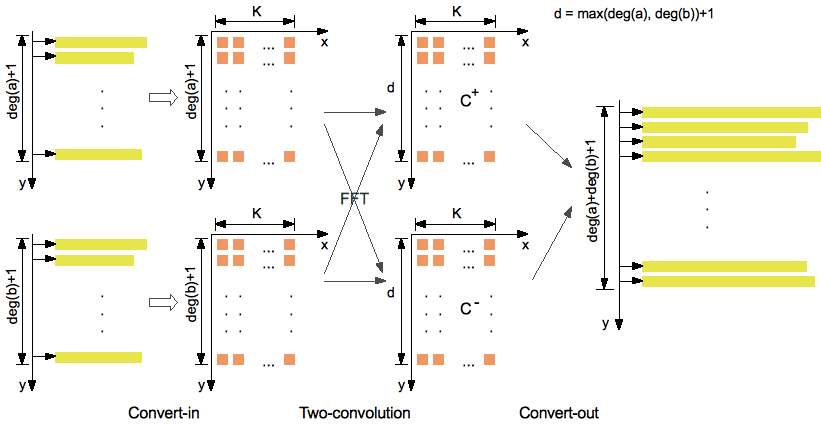Notations:
• F(e,s) is an upper bound of the number of bot operations for computing the FFT of a vector of size s over a prime field Ζ/p with e Θ(log2(p)).
• If p is a Fourier prime, we have F(e,s) Θ(s  log2(s)  e  log2(e)  log2log2(e)).
• If e Θ(log2(s)) and p is a generalized Fermat prime, then F(e,s) Θ(s  log2(s)  e  log2log2(e)).
Assumptions (mild):
• K Θ(d)
• M Θ(log2(d))
Algebraic complexity (work, bit ops):
• if p is a Fourier prime, then Ο(d2  log22(d)  log2log2log2(d)) (implemented and available)
• if p is a generalized Fermat prime, then Ο(d2  log2(d)  log2log2log2(d)) (work in progress)
• in comparison, Schönaghe-Strassen gives here Ο(d2  log2(d)  log2log2(d))
Parallel complexity (span, bit ops): Ο(log23(d)  log2log2log2(d))
Our code relies only and directly on machine word operations (addition, shift and multiplication).
• Experimental results are on a 48-core AMD Opteron 6168 node: BPAS (parallel) vs FLINT (serial) vs Maple 2015 (parallel)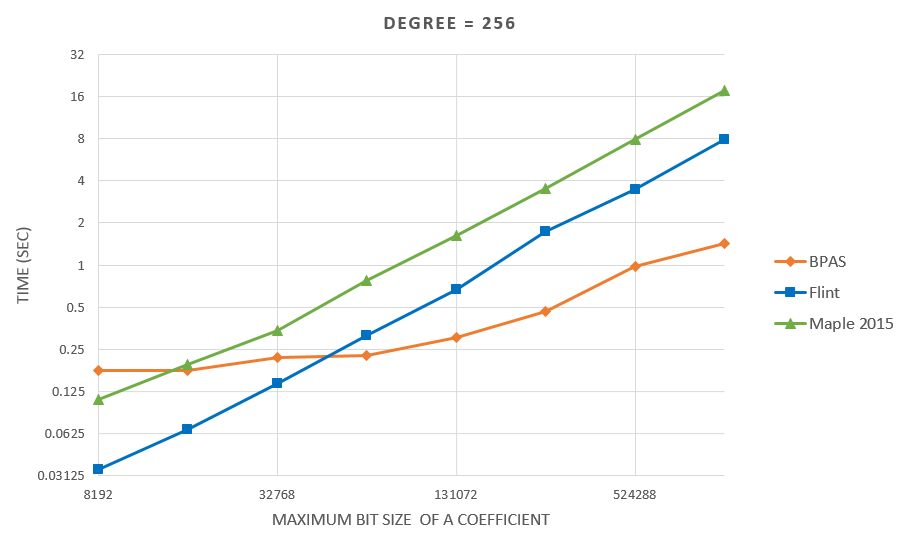## Symbolic-numeric integration

Multiprecision approximate integration of rational functions with error control using two methods:

1) Exact integration followed by a numerical approximation of the integral:

• Hermite reduction (rational part) and Lazard-Rioboo-Trager algorithm (transcendental part) to integrate;
• Multiprecision real-root isolation on factors of the resultant using MPSolve;
• Symbolic post-processing to compute arctangent terms.
• Comparison between 53-bit and 106-bit precisions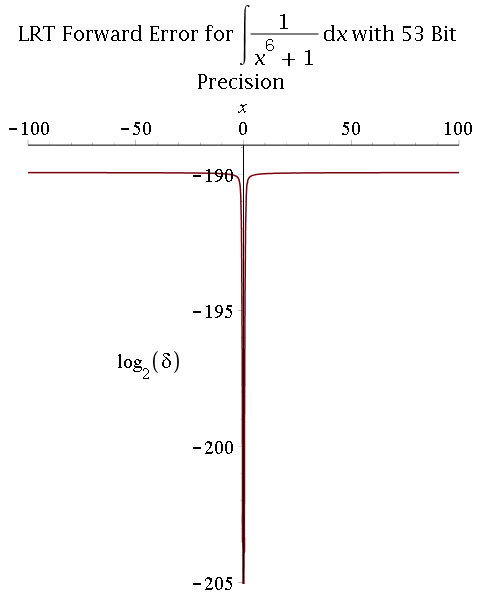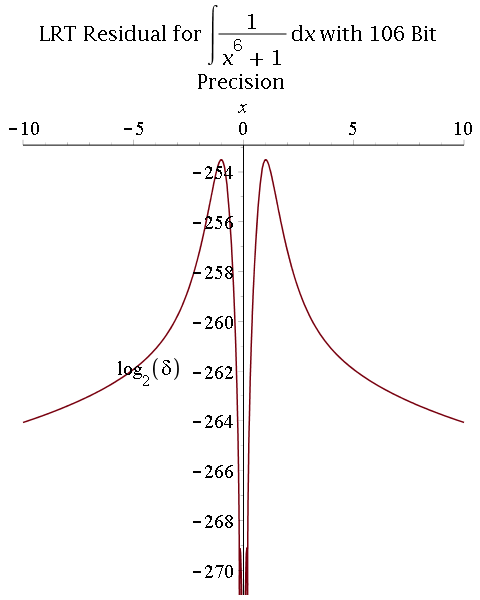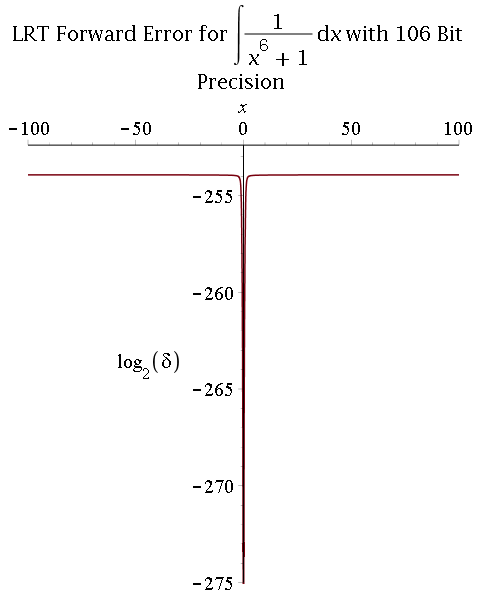2) Multiprecision partial fraction decomposition (PFD) followed by exact integration (proposed by R. Fateman):

• Multiprecision real-root isolation for denominator using MPSolve;
• Symbolic computation of partial fraction decomposition of reconstructed denominator;
• Term-wise integration of rational terms and linear and quadratic (denominator) transcendental terms.
• Comparison between 53-bit and 106-bit precisions: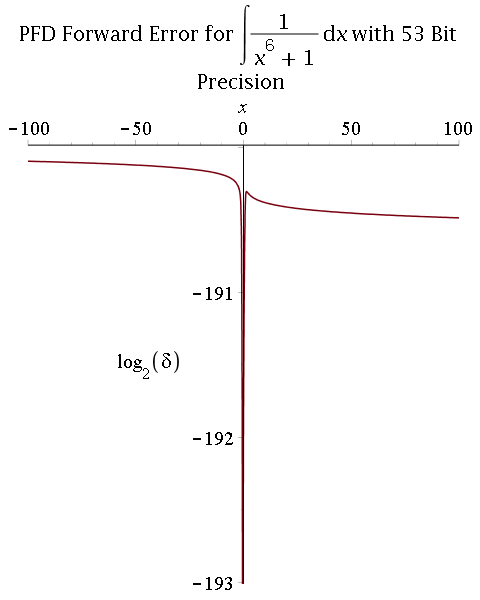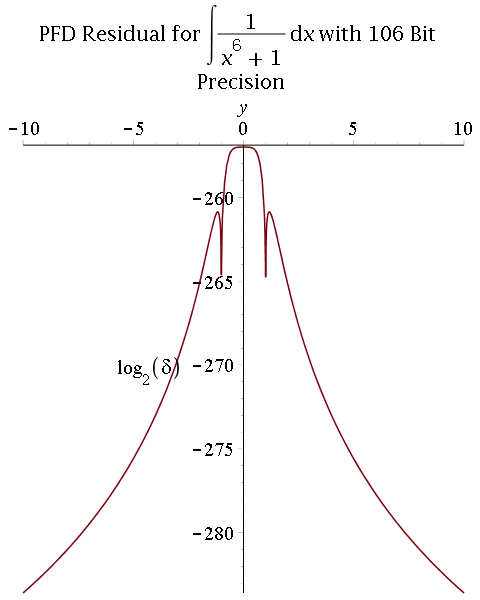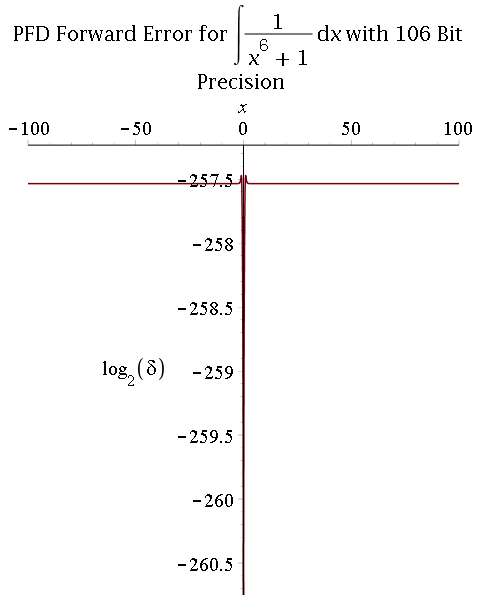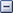Parallel real root isolation

### Parallel univariate real root isolation

• Experimental results: Running time (in sec.) on a 48-core AMD Opteron 6168 node for BPAS (parallel) vs CMY (parallel) vs Maple 18 realroot (serial)### Parallel multivariate real root isolation

• Experimental results: Running time (in sec.) on a 12-core Intel Xeon 5650 node for BPAS (parallel) vs. Maple 17 RealRootIsolate (serial) vs. C (with Maple 17 interface) Isolate (serial)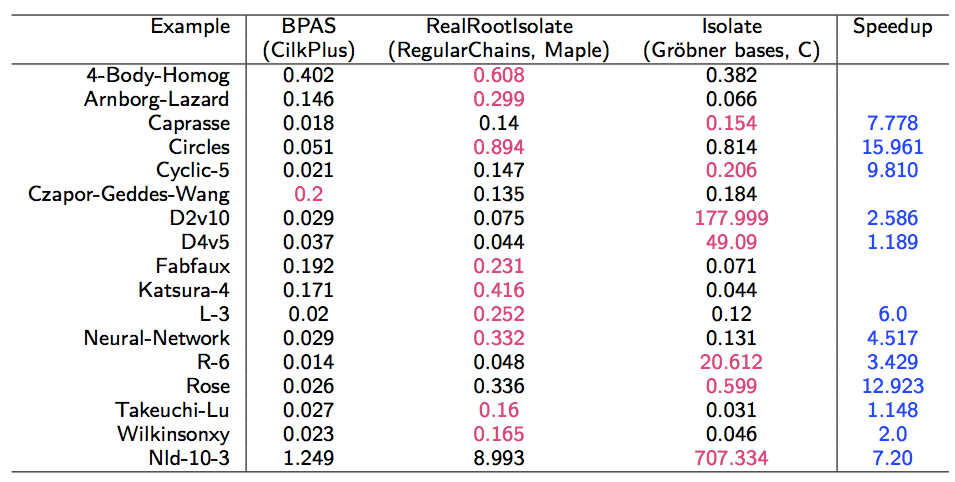ORCCA Lab, Department of Computer Science, The University of Western Ontario, London, Ontario, Canada N6A 5B7